## Example Questions

### Example Question #41 : Statistics

A thermometer reads an average of 47.5 °F on a Sunday, rises 2.9 degrees on Monday, and drops 1.7 degrees on Tuesday. What is the average reading of the thermometer on Tuesday?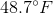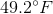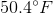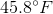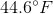Explanation:

Use 47.5 as a baseline value to add and subtract the subsequent drops on Monday and Tuesday. Thus, 47.5 + 2.9 = 50.4 (Monday); 50.4 - 1.7 = 48.7 (Tuesday).

### Example Question #42 : Statistics

What is the sum of the mean and the median of the following set of numbers:

5, 12, 7, 28, 8?

None of the answers are correct

12

24

20

8

20

Explanation:

First, put the data in ascending order:  5, 7, 8, 12, 28

Mean = average = (5 + 7 + 8 + 12 + 28) ÷ 5 = 60 ÷ 5 = 12

Median = middle number = 8

Mean + Median = 12 + 8 = 20

### Example Question #43 : Statistics

Samantha’s semester math grade is based on the average of five unit tests.  She has already achieved test scores of 88, 92, 79, and 95.  What does she need to score on her next test to obtain a 90 as the final grade for the semester?

90

96

89

85

None of the answers are correct

96

Explanation:

To get an average of 90 for the five tests, she must score a total of 450 points (90 x 5).  If you add up the first four test scores, the total is 354 points (88 + 92 + 79 + 95).  Subtracting where she is from where she wants to be (450 – 354), you get a fifth test score of 96.

### Example Question #41 : How To Find Arithmetic Mean

Jill has received $200 on her birthday each year for four consecutive years. For the next two years she received$700 in total. For this 6-year period, what was her average yearly amount of birthday money she received?

$350$250

$275$200

$400 Correct answer:$250

Explanation:

We add $200 times the four years, giving us$800. We then add $700 for the following two years, giving a total of$1,500. We divide this by 6 years, giving us an average of \$250 per year.

### Example Question #41 : Arithmetic Mean

Find the arithmetic mean of the following set of data:

{1,2,3,4,6,8}Explanation:

The total sum of the elements of the set is 24. There are 6 terms in the set.

24/6 = 4

### Example Question #52 : Data Analysis

The following are the points scored on a test by students in a class:

5,9,11,12,13,13,18,20

Which of the following is closest to the mean?

12

13

14

11

15

13

Explanation:

13 is the correct answer. The mean is all the scores divided by the number of scores, which in this case is 12.625.

### Example Question #42 : Statistics

Find the arithmetic mean of this set of data:

{0, 0, 0, 0, 1, 5}

3

0

1

5

4

1

Explanation:

The sum of all the terms is 6. The number of terms in the set is 6. 6/6 = 1

### Example Question #42 : How To Find Arithmetic Mean

Greg got an average of 93 on his test scores this semester. He got a 92, 93, and 97 on the first three tests. If he received the same score on each of his last three tests, what was his score on each of these tests?

89

92

94

90.5

92.33

92

Explanation:

We can set up an equation (92 + 93 + 97 + 3x)/6 = 93. Solving for x yields 92.

### Example Question #41 : Arithmetic Mean

Tom needs an average test score of 75 to pass his math class. The class gives five equally-weighted tests, and his first four scores were 57, 63, 90, and 72. What score does he need to receive on his final test to pass his class?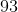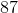Explanation:

The formula for the average of Tom's scores is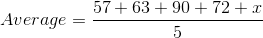He needs an average of 75, so his average is represented by the following formula where "" represents his fifth test score.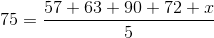Multiply the five across: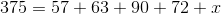Subtracting: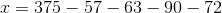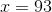### Example Question #11 : Algebraic Fractions

If the average (arithmetic mean) of,, andis, what is the average of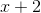,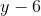, and?There is not enough information to determine the answer.Explanation:

If we can find the sum of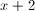,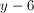, and 10, we can determine their average. There is not enough information to solve foror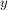individually, but we can find their sum,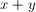Write out the average formula for the original three quantities.  Remember, adding together and dividing by the number of quantities gives the average: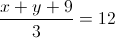Isolate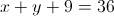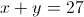Write out the average formula for the new three quantities: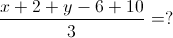Combine the integers in the numerator: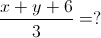Replacewith 27: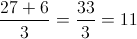### All ACT Math Resources